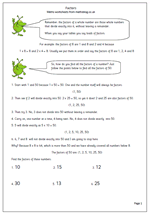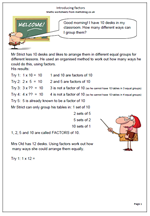## Year 6 resource of the week: factorsUnderstanding factors and multiples is something many children fail to grasp. Here we have a page on factors and how to find all the factors of a number. The process is fairly straightforward, but can be quite time consuming. A good knowledge of tables and division is also needed. So if children’s knowledge of tables is weak, if they find division difficult and if they have little staying power then it is unlikely that they will enjoy trying to find factors of numbers!

One point that even brighter children take a while to understand is that you only need to continue to divide up to when the number squared is larger than the original number eg to find the factors of 62 you only need to divide 62 by numbers up to 7 because eight eights are 64. It is a good idea to spend some time explaining and showing the logic of this to children.

This can be found in our Year 6 Understanding Number section.

Factors 1

## Understanding factorsUnderstanding factors can prove to be quite tricky, but in fact is a fairly simple concept, with once again knowing times tables being crucial. These pages begin by trying to find the factors of a number on an organised ‘trial and error’ basis by seeing which numbers when multiplied together will make the target number.

When doing this it saves time if it is understood when to stop.

For example; the factors of 10:

Try 1:  1 x 10 =  10        1 and 10 are factors of 10
Try 2:  2 x  5  =  10        2 and 5 are factors of 10
Try 3:  3 x ??  =  10       3 is not a factor of 10 (so he cannot have 10 tables in 3 equal groups)
Try 4:  4 x ??  =  10       4 is not a factor of 10 (so he cannot have 10 tables in 4 equal groups)
Try 5:  5 is already known to be a factor of 10 2 x 5 is the same as 5 x 2. There is no need to go further than this.

Introducing factors

## Year 6 maths worksheet: FactorsUnderstanding factors and multiples is something many children fail to grasp. Here we have a page on factors and how to find all the factors of a number. The process is fairly straightforward, but can be quite time consuming. A good knowledge of tables and division is also needed. So if children’s knowledge of tables is weak, if they find division difficult and if they have little staying power then it is unlikely that they will enjoy trying to find factors of numbers!

One point that even brighter children take a while to understand is that you only need to continue to divide up to when the number squared is larger than the original number eg to find the factors of 62 you only need to divide 62 by numbers up to 7 because eight eights are 64. It is a good idea to spend some time explaining and showing the logic of this to children.

This can be found in our Year 6 Understanding Number section.

Factors 1